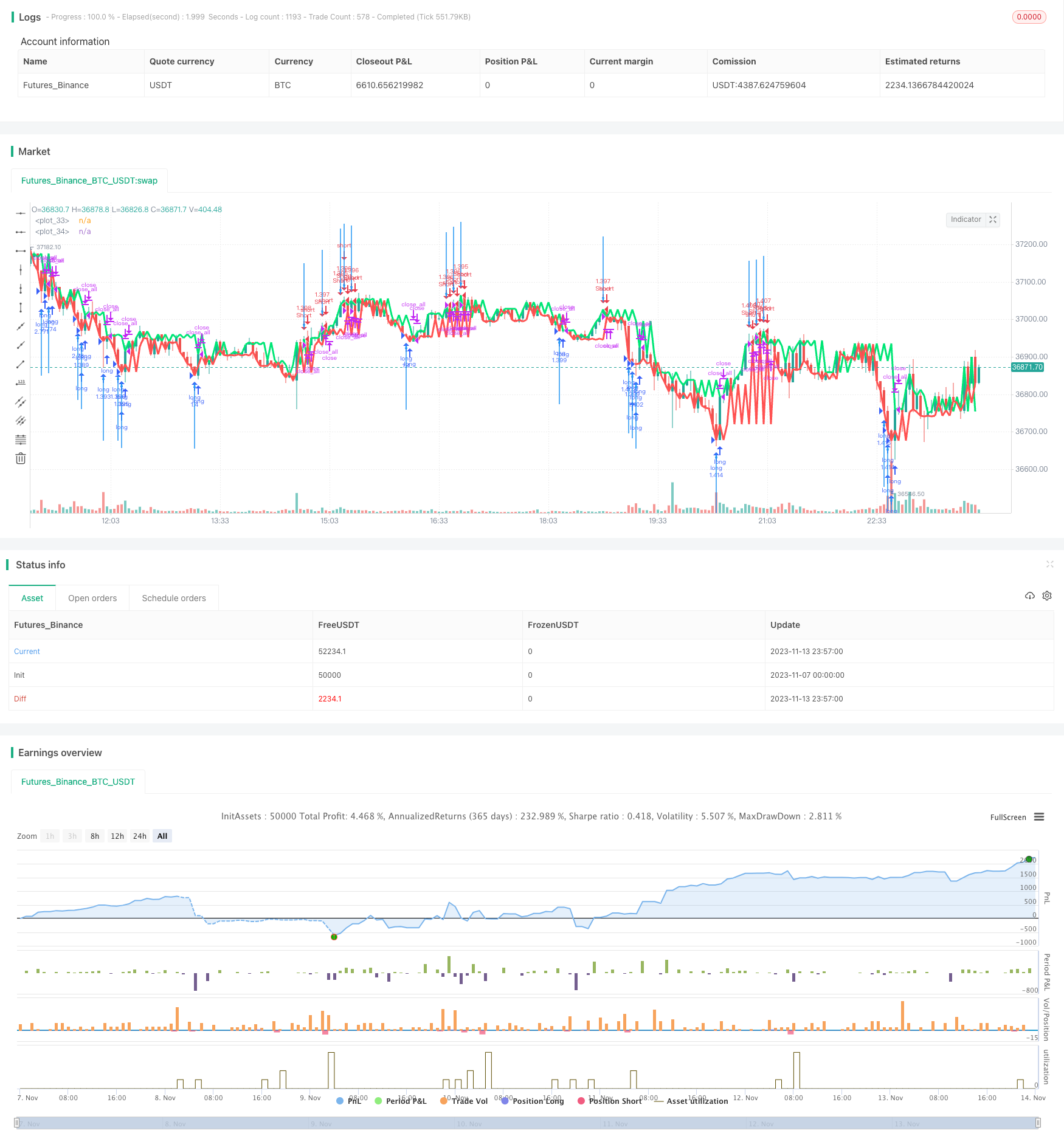# Trend Following Strategy Based on Distance with Trailing Stop Loss

Author: ChaoZhang, Date: 2023-11-15 11:24:16
Tags:## Overview

This strategy utilizes the Distance Close Bars (DCB) indicator to determine price trend and fast RSI indicator as a filter, implements trailing stop loss for trend following trading. It also uses martingale principle for position sizing. Suitable for medium-long term trend trading.

## Principles

1. Calculate lastg and lastr representing last green bar’s close and last red bar’s close.

2. Calculate dist as the difference between lastg and lastr.

3. Calculate adist as 30-period SMA of dist.

4. Generate trading signal when dist is greater than 2 times of adist.

5. Use fast RSI indicator to filter signal, avoiding false breakout.

6. Enter trade at fixed percentage of equity if signal presents with no position.

7. Martingale to scale in after loss.

8. Close position when stop loss or take profit is triggered.

1. DCB indicator effectively captures mid-long term trends.

2. Fast RSI filter avoids losses from false breakouts.

3. Trailing stop locks in profits and controls risks.

4. Martingale increases position after loss for higher profit.

5. Reasonable parameter settings suit different market environments.

## Risks

1. DCB may generate wrong signals, needs other filters.

2. Martingale can amplify losses, requires strict risk management.

3. Improper stop loss setting may lead to excessive loss.

4. Position sizing should be limited to prevent over-leverage.

5. Improper contract settings may lead to huge loss in extreme market.

## Optimization

1. Optimize DCB parameters for best combination.

2. Try other indicators to replace fast RSI filter.

3. Optimize stop loss and take profit for higher win rate.

4. Optimize martingale parameters to reduce risk.

5. Test on different products for best asset allocation.

6. Use machine learning to dynamically optimize parameters.

## Summary

This is an overall mature trend following strategy. DCB determines trend direction and fast RSI filters signals to avoid wrong entries. Stop loss and take profit effectively controls single trade loss. But there are still risks, parameters need further optimization to reduce risk and improve stability. The logic is clear and easy to understand, suitable for mid-long term trend traders.

```/*backtest
start: 2023-11-07 00:00:00
end: 2023-11-14 00:00:00
period: 3m
basePeriod: 1m
exchanges: [{"eid":"Futures_Binance","currency":"BTC_USDT"}]
*/

//Noro
//2018

//@version=2
strategy(title = "Noro's Distance Strategy v1.0", shorttitle = "Distance str 1.0", overlay = true, default_qty_type = strategy.percent_of_equity, default_qty_value = 100, pyramiding = 10)

//Settings
needlong = input(true, defval = true, title = "Long")
needshort = input(true, defval = true, title = "Short")
usemar = input(true, defval = true, title = "Use Martingale")
capital = input(100, defval = 100, minval = 1, maxval = 10000, title = "Capital, %")
usersi = input(true, defval = true, title = "Use RSI-Filter")
periodrsi = input(7, defval = 7, minval = 2, maxval = 50, title = "RSI Period")
limitrsi = input(30, defval = 30, minval = 1, maxval = 50, title = "RSI Limit")
fromyear = input(2018, defval = 2018, minval = 1900, maxval = 2100, title = "From Year")
toyear = input(2100, defval = 2100, minval = 1900, maxval = 2100, title = "To Year")
frommonth = input(01, defval = 01, minval = 01, maxval = 12, title = "From Month")
tomonth = input(12, defval = 12, minval = 01, maxval = 12, title = "To Month")
fromday = input(01, defval = 01, minval = 01, maxval = 31, title = "From day")
today = input(31, defval = 31, minval = 01, maxval = 31, title = "To day")

//Fast RSI
fastup = rma(max(change(close), 0), periodrsi)
fastdown = rma(-min(change(close), 0), periodrsi)
fastrsi = fastdown == 0 ? 100 : fastup == 0 ? 0 : 100 - (100 / (1 + fastup / fastdown))

//Distance
bar = close > open ? 1 : close < open ? -1 : 0
lastg = bar == 1 ? close : lastg
lastr = bar == -1 ? close : lastr
dist = lastg - lastr
plot(lastg, linewidth = 3, color = lime)
plot(lastr, linewidth = 3, color = red)
up = bar == -1 and dist > adist * 2
dn = bar == 1 and dist > adist * 2

//RSI Filter
rsidn = fastrsi < limitrsi or usersi == false
rsiup = fastrsi > 100 - limitrsi or usersi == false

//Signals
up1 = up and rsidn
dn1 = dn and rsiup
exit = ((strategy.position_size > 0 and close > open) or (strategy.position_size < 0 and close < open))

//Arrows
plotarrow(up1 ? 1 : na, colorup = blue, colordown = blue)
plotarrow(dn1 ? -1 : na, colorup = blue, colordown = blue)

profit = exit ? ((strategy.position_size > 0 and close > strategy.position_avg_price) or (strategy.position_size < 0 and close < strategy.position_avg_price)) ? 1 : -1 : profit
mult = usemar ? exit ? profit == -1 ? mult * 2 : 1 : mult : 1
lot = strategy.position_size == 0 ? strategy.equity / close * capital / 100 * mult : lot

signalup = up1
if signalup
if strategy.position_size < 0
strategy.close_all()

strategy.entry("long", strategy.long, needlong == false ? 0 : lot, when=(time > timestamp(fromyear, frommonth, fromday, 00, 00) and time < timestamp(toyear, tomonth, today, 23, 59)))

signaldn = dn1
if signaldn
if strategy.position_size > 0
strategy.close_all()

strategy.entry("Short", strategy.short, needshort == false ? 0 : lot, when=(time > timestamp(fromyear, frommonth, fromday, 00, 00) and time < timestamp(toyear, tomonth, today, 23, 59)))

if time > timestamp(toyear, tomonth, today, 23, 59) or exit
strategy.close_all()
```

More